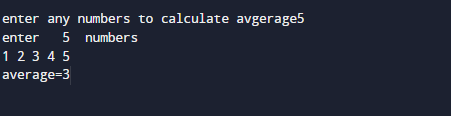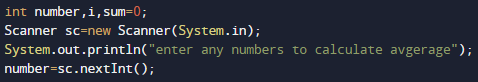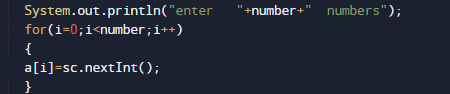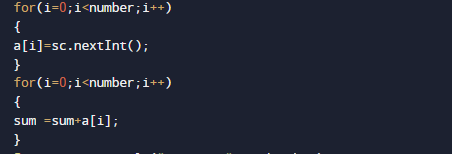# Java Program to Calculate Average Of N Numbers

In this tutorial you will learn about the Java Program to Calculate Average Of N Numbers and its application with practical example.

In this tutorial, we will learn to create a Java Program to Calculate Average Of N Numbers using Java programming.

## Prerequisites

Before starting with this tutorial we assume that you are best aware of the following Java programming topics:

• Java Operators.
• Basic Input and Output function in Java.
• Class and Object in Java.
• Basic Java programming. Basic Java programming.
• If-else statements in Java.
• For loop in Java.

## Average of  numbers.

Average is the sum of all data values Dived by the Number of data values.

Average=sum of n natural number divided by N or  “[n(n+1)/2]”.

## Java Program to Calculate Average Of N Numbers

In this program we will learn to create a program to calculate Average of N number. Firstly we declare required header file and variable and also initiates required values in variable. Next we take value from user at run time and then after we will find the Average of N natural number.

## OutputIn our program with the help of for loops we loop from 1 to the given number (5) and add all numbers to the variable Sum.
sum=sum+i. and after we calculate average of values “average=sum/number“.In the above program, we have first declared and initialized a set variables required in the program.

• number=to take value from user.
• i =For iteration.
• sum = for counting sum of numbers.Here we first declared and initialized set of variables required in the program. Then we will take a value from user.After that we will start loop from 1 to given number to add values in array.after taking these values we will add these values in loop.Within the loop, we add all values in variable Sum .After calculating sum of values of N natural number between the given numbers natural number we find average of n values and print the result in output.In this tutorial we have learn about the Java Program to Calculate Average Of N Numbers and its application with practical example. I hope you will like this tutorial.